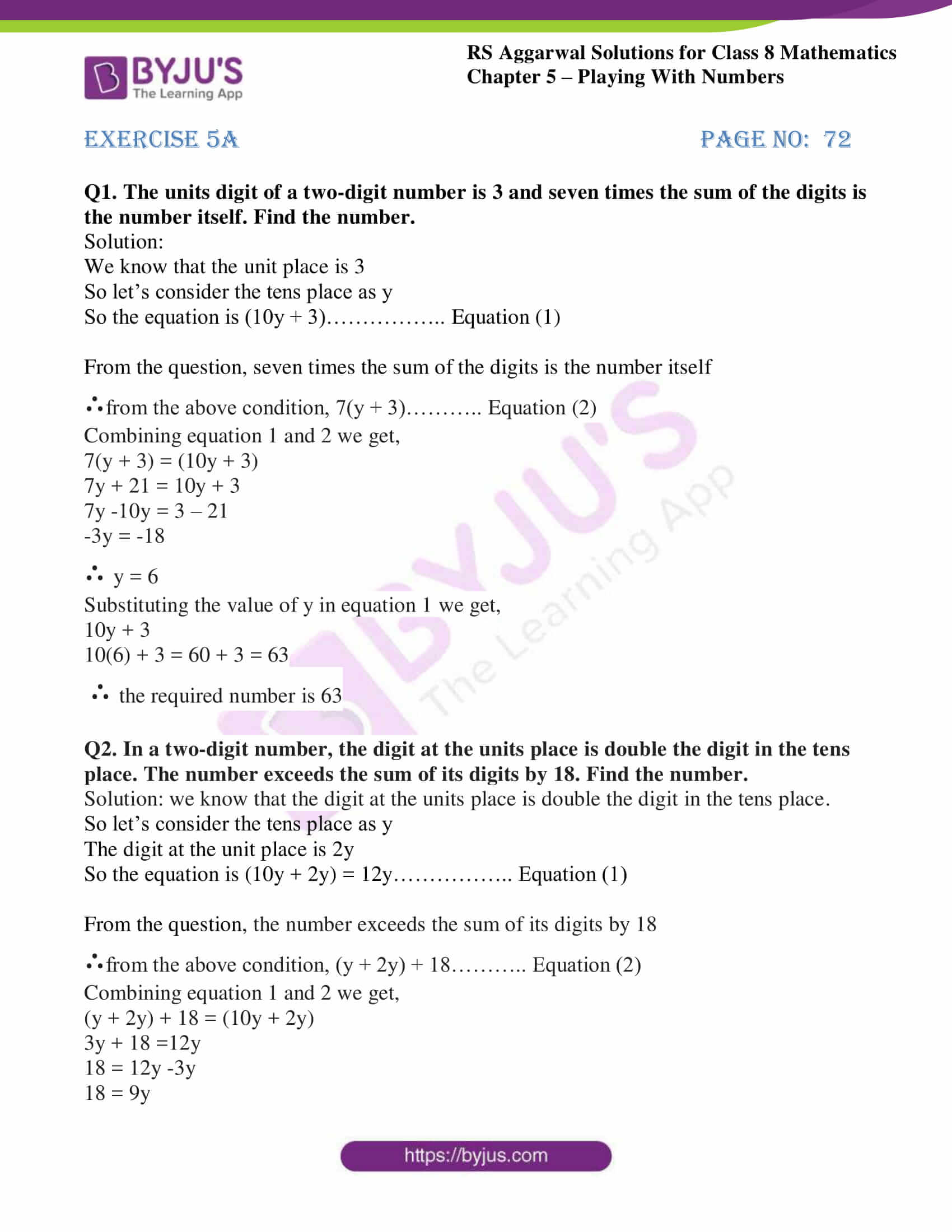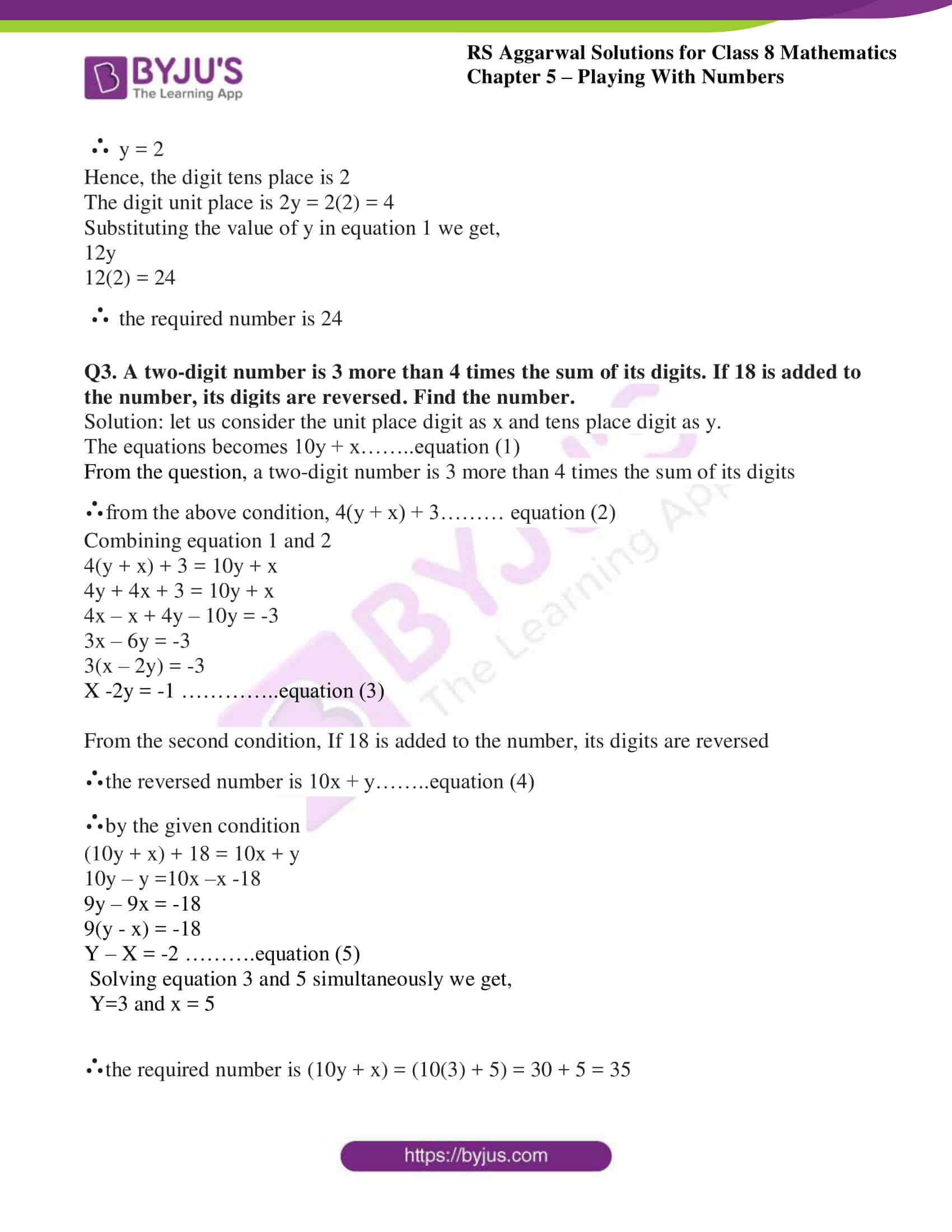# RS Aggarwal Solutions for Class 8 Maths Chapter 5 - Playing with Numbers Exercise 5A

Students can refer and download RS Aggarwal Solutions for Class 8 Maths Chapter 5- Exercise 5A, Playing with Numbers from the links provided below. Our experts have solved the RS Aggarwal Solutions to ensure that the students have in-depth knowledge of the basic concepts by practicing the solutions.

In Exercise 5A of RS Aggarwal Class 8 Maths, we shall study Numbers in the generalised form- A number is said to be in a generalised form if it is expressed as the sum of the products of its digits with their respective place values.

## Download PDF of RS Aggarwal Solutions for Class 8 Maths Chapter 5- Playing with Numbers Exercise 5A### Access answers to RS Aggarwal Solutions for Class 8 Maths Chapter 5- Playing with Numbers Exercise 5A

Q1. The units digit of a two-digit number is 3 and seven times the sum of the digits is the number itself. Find the number.

Solution:

We know that the unit place is 3

So let’s consider the tens place as y

So the equation is (10y + 3)…………….. Equation (1)

From the question, seven times the sum of the digits is the number itself

∴from the above condition, 7(y + 3)……….. Equation (2)

Combining equation 1 and 2 we get,

7(y + 3) = (10y + 3)

7y + 21 = 10y + 3

7y -10y = 3 – 21

-3y = -18

∴ y = 6

Substituting the value of y in equation 1 we get,

10y + 3

10(6) + 3 = 60 + 3 = 63

∴ the required number is 63

Q2. In a two-digit number, the digit at the units place is double the digit in the tens place. The number exceeds the sum of its digits by 18. Find the number.

Solution: we know that the digit at the units place is double the digit in the tens place.

So let’s consider the tens place as y

The digit at the unit place is 2y

So the equation is (10y + 2y) = 12y…………….. Equation (1)

From the question, the number exceeds the sum of its digits by 18

∴from the above condition, (y + 2y) + 18……….. Equation (2)

Combining equation 1 and 2 we get,

(y + 2y) + 18 = (10y + 2y)

3y + 18 =12y

18 = 12y -3y

18 = 9y

∴ y = 2

Hence, the digit tens place is 2

The digit unit place is 2y = 2(2) = 4

Substituting the value of y in equation 1 we get,

12y

12(2) = 24

∴ the required number is 24

Q3. A two-digit number is 3 more than 4 times the sum of its digits. If 18 is added to the number, its digits are reversed. Find the number.

Solution: let us consider the unit place digit as x and tens place digit as y.

The equations becomes 10y + x……..equation (1)

From the question, a two-digit number is 3 more than 4 times the sum of its digits

∴from the above condition, 4(y + x) + 3……… equation (2)

Combining equation 1 and 2

4(y + x) + 3 = 10y + x

4y + 4x + 3 = 10y + x

4x – x + 4y – 10y = -3

3x – 6y = -3

3(x – 2y) = -3

x -2y = -1 …………..equation (3)

From the second condition, If 18 is added to the number, its digits are reversed

∴the reversed number is 10x + y……..equation (4)

∴by the given condition

(10y + x) + 18 = 10x + y

10y – y =10x –x -18

9y – 9x = -18

9(y – x) = -18

y – x = -2 ……….equation (5)

Solving equation 3 and 5 simultaneously we get,

y=3 and x = 5

∴the required number is (10y + x) = (10(3) + 5) = 30 + 5 = 35

### Access other Exercises of RS Aggarwal Solutions for Class 8 Maths Chapter 5- Playing with Numbers

Exercise 5B Solutions 15 Questions

Exercise 5C Solutions 14 Questions

Exercise 5D Solutions 9 Questions

## RS Aggarwal Solutions for Class 8 Maths Chapter 5- Playing with Numbers Exercise 5A

Exercise 5A of RS Aggarwal Class 8, Playing with Numbers contains the basic concepts related to Numbers. This exercise mainly deals with the basic properties of numbers. Some of them include

• Two-digit Numbers.
• Three-digit Numbers.

The RS Aggarwal Solutions can help the students be rigorous in their practice while learning the fundamentals as it provides all the answers to the questions from the RS Aggarwal textbook. Students are suggested to practice the problems on a regular basis which will help them excel in their exams and increase their overall percentage. Practicing more number of times helps in achieving high marks, in turn, helps in time management skills and also improves the confidence level.